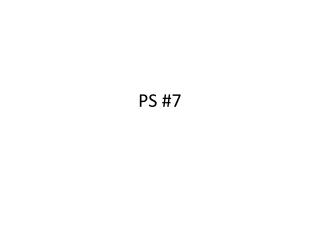DownloadDownload PresentationPS #7

# PS #7

Télécharger la présentation## PS #7

- - - - - - - - - - - - - - - - - - - - - - - - - - - E N D - - - - - - - - - - - - - - - - - - - - - - - - - - -
##### Presentation Transcript

1. PS #7

2. SD Worksheet

3. Perception of Bush, Clinton, and Perot Ideological Placements, 1992 • Range for each chart? • Greatest/Smallest Dispersion/SD? • Minimum Possible Dispersion/SD? • Maximum Possible Dispersion/SD?

4. SD of Seasonal Snowfall • The four highlighted years deviate upwards from the mean of 15.2" by about by about 23, 16, 31, and 25 inches. • The four other above normal years deviate upwards by an average amount of about 10. • So the sum of the positive deviations is about 23 + 16 + 31 + 25 + 30 = 125 inches. • It appears that average snowfall in the 19 below normal years is about 10 inches, for an average negative deviation of about 5 inches. • So the sum of the negative deviations is about −95 inches. • There are also three years when snowfall was almost exactly “normal.” So the sum of the absolute deviations is about 120, and the mean deviation is about 120/29 ≈4 inches. The standard deviation is typically somewhat lager than the mean deviation, so the SD is about 5 to 6 inches.